Up: Functional Dependencies Previous: Closure of Attribute Sets

Canonical Cover

1. To minimize the number of functional dependencies that need to be tested in case of an update we may restrict F to a canonical cover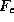.
2. A canonical cover for F is a set of dependencies such that F logically implies all dependencies in, and vice versa.
3.must also have the following properties:
• Every functional dependency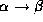incontains no extraneous attributes in(ones that can be removed fromwithout changing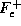). So A is extraneous inif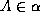and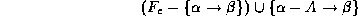logically implies.

• Every functional dependencyincontains no extraneous attributes in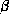(ones that can be removed fromwithout changing). So A is extraneous inif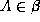and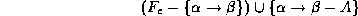logically implies.

• Each left side of a functional dependency inis unique. That is there are no two dependenciesand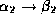insuch that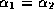.
4. To compute a canonical coverfor F,

repeat

Use the union rule to replace dependencies of the formand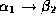with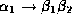.

Find a functional dependencywith an

extraneous attribute inor in.

If an extraneous attribute is found, delete it fromuntil F does not change.

5. An example: for the relational scheme R=(A,B,C), and the set F of functional dependencies

ABC

BC

AB

ABC

we will compute.

• We have two dependencies with the same left hand side:

ABC

AB

We can replace these two with just ABC .

• A is extraneous in ABC because BC logically implies ABC .

• Then our set is

ABC

BC

• We still have an extraneous attribute on the right-hand side of the first dependency. C is extraneous in ABC because AB and BC logically imply that ABC .
• So we end up with

AB

BC